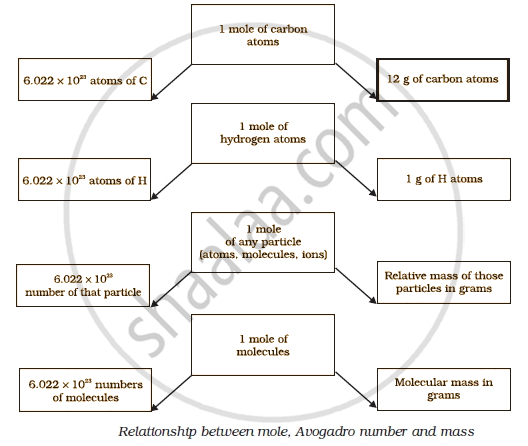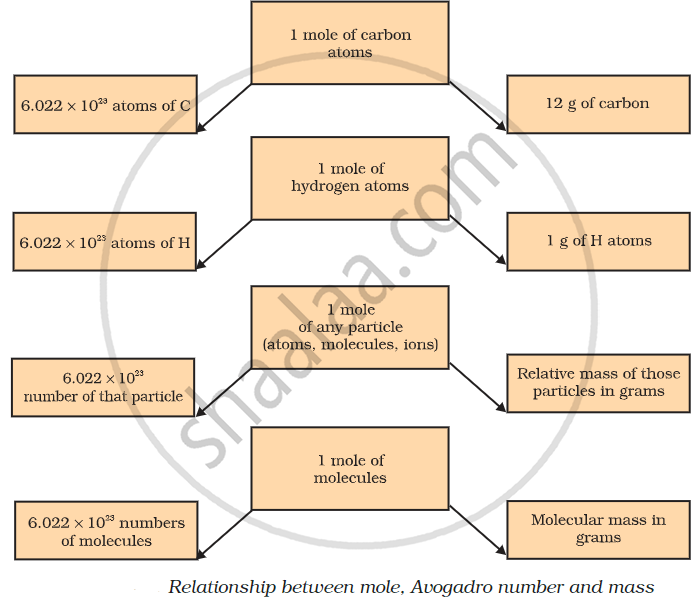Tamil Nadu Board of Secondary EducationSSLC (English Medium) Class 10th

# Mole Concept

## Notes

Take an example of the reaction of hydrogen and oxygen to form water:

2H2+ O2 → 2H2O.

The above reaction indicates that
(i) two molecules of hydrogen combine with one molecule of oxygen to form two molecules of water, or
(ii) 4 u of hydrogen molecules combine with 32 u of oxygen molecules to form 36 u of water molecules.

We can infer from the above equation that the quantity of a substance can be characterised by its mass or the number  of molecules. But, a chemical reaction equation indicates directly the number of atoms or molecules taking part in the reaction. Therefore, it is more convenient to refer to the quantity of a substance in terms of the number of its molecules or atoms, rather than their masses. So, a new unit “mole” was introduced. One mole of any species (atoms,molecules, ions or particles) is that quantity in number having a mass equal to its atomic or molecular mass in grams.

The number of particles (atoms, molecules or ions) present in 1 mole of any substance is fixed, with a value of 6.022 ×  1023. This is an experimentally obtained value. This number is called the Avogadro Constant or Avogadro Number (represented by N0),named in honour of the Italian scientist, Amedeo Avogadro. 1 mole (of anything) = 6.022 × 1023 in number,

as, 1 dozen = 12 nos.
1 gross = 144 nos.

Besides being related to a number, a mole has one more advantage over a dozen or a gross. This advantage is that mass of 1 mole of a particular substance is also fixed.

The mass of 1 mole of a substance is equal to its relative atomic or molecular mass in grams. The atomic mass of an lement gives us the mass of one atom of that element in atomic mass units (u). To get the mass of 1 mole of atom of that element, that is, molar mass, we have to take the same numerical value but change the units from ‘u’ to ‘g’. Molar
mass of atoms is also known as gram atomic mass. For example, atomic mass of hydrogen=1u. So, gram atomic mass of hydrogen = 1 g.

## Notes

### Mole:

It is a method of expressing the amount of a substance.

Take a pause and remember the various units of measurement.

Now, consider a human with a weight of 60Kgs. Why did we express it in Kgs and not in meters?

So basically, every measurement has magnitude with its SI unit. In this case, 60 was the magnitude and the SI unit was Kgs!

Atomically, we can measure the weight with the help of this mole concept.

Your body weight is inclusive of all the cells present inside your body. Likewise, One gram of element will have many atoms and its mass will be inclusive of all of them!

Like we discussed their molecular mass, now we will measure it in moles.

A mole is the chemist’s counting unit and 1 mole is equivalent to 6.022 × 1023 number.

Now let’s start with the denotation of the various term that will help us in solving the numerical -

 Denoted by SI unit Given mass m Grams (g) Molar mass M Grams (g) Given the number of particles N - Avogadro number of particles N0 - Number of moles n Moles

Formula:

"no. of moles" = "given mass"/"molar mass" = "given number of particles"/"given number of particles"

"n" = "m"/"M" = "N"/N_0If you would like to contribute notes or other learning material, please submit them using the button below.

### Shaalaa.com

Atoms and Molecules (Mole Concepts) [00:22:07]
S
0%